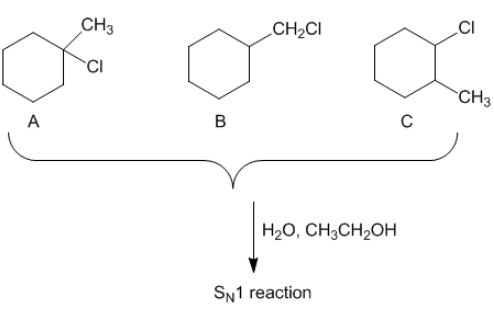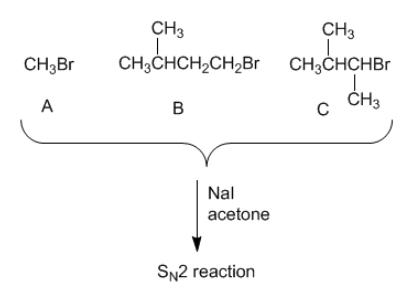# Problem: A set of three nucleophilic displacement reactions is shown below:Which reaction (A, B, or C) proceeds the fastest?A set of three nucleophilic displacement reactions is shown below:Which reaction (A, B, or C) proceeds the fastest?Which reaction (A, B, or C) proceeds the slowest?

###### FREE Expert Solution

For this problem, we have to determine which of the reaction will be the fastest or slowest for each SN1 and SN2 reaction

Recall that when given a substitution reaction, we will use the following two factors to determine the mechanism:

Nucleophile Strength:              SN1 =   WEAK               SN2 = STRONG

Leaving Group Substitution:    SN = 3° > 2°                 SN2 = 0° > 1° > 2°

Let's analyze each problem:

81% (57 ratings)###### Problem Details

A set of three nucleophilic displacement reactions is shown below:Which reaction (A, B, or C) proceeds the fastest?

A set of three nucleophilic displacement reactions is shown below:Which reaction (A, B, or C) proceeds the fastest?

Which reaction (A, B, or C) proceeds the slowest?

Frequently Asked Questions

What scientific concept do you need to know in order to solve this problem?

Our tutors have indicated that to solve this problem you will need to apply the Substitution Comparison concept. You can view video lessons to learn Substitution Comparison. Or if you need more Substitution Comparison practice, you can also practice Substitution Comparison practice problems.

What is the difficulty of this problem?

Our tutors rated the difficulty ofA set of three nucleophilic displacement reactions is shown ...as medium difficulty.

What professor is this problem relevant for?

Based on our data, we think this problem is relevant for Professor Walker's class at MSU.